×

Search anything:

# Euler’s Polyhedron Formula

#### computational geometry Algorithms List of Mathematical AlgorithmsGet this book -> Problems on Array: For Interviews and Competitive Programming

In this article, we have explored Euler’s Polyhedron Formula in depth with examples, proof and applications in real life problems.

1. Introduction to Euler’s Polyhedron Formula
2. Examples
3. Euler’s Characteristic
4. Mathematical Proof and converting into Network
5. Application of Euler’s Polyhedron Formula

# Introduction to Euler’s Polyhedron Formula

Euler’s Polyhedron formula states that for all convex Polyhedrons, if we add all the number of faces in a polyhedron, with all the number of polyhedron vertices, and then subtract all the number of polyhedron edges, we always get the number two as a result.

The formula in mathematical terms is as follows-
F+V-E = 2,

where F, V and E stand for the number of faces, vertices and edges in a polyhedron.

To better understand this formula, we need to understand polyhedrons in general.

A polyhedron is a 3d shape that has flat polygonal faces. Lines joining these faces are known as the edges. In addition, we call the corners of these polygonal faces the vertices.

Euler’s formula works for all convex Polyhedrons. For a convex polyhedron, all its diagonals must be inside its structure. Polyhedrons should not have holes in their structure. We consider such polyhedrons as valid applicants for Euler’s formula. In addition, valid Polyhedrons cannot have separate parts, where only one shared edge or vertex exists. However, they are some exclusions as well. Certain non-convex polyhedrons can also produce the same results as Euler’s formula.

# Examples

### Cube

To further understand Euler’s formula, we can take the example of a cube.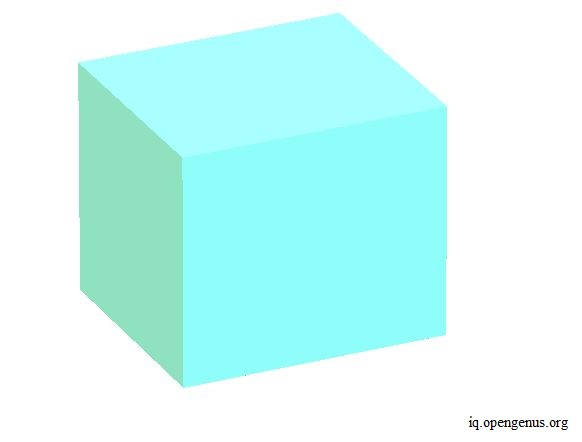A cube has six faces- top, bottom, left, right, front and back. Counting the corner of all these faces gives us eight vertices. Joining all the faces, we get 12 edges. So according to the formula we get,

6+8-12 = 2

As we can observe from above, adding the number of vertices and faces together, and then subtracting the number edges from the resultant sum, gives us the number two.

### Tetrahedron

We can also consider a Tetrahedron. A Tetrahedron has a triangular face at the bottom and three more triangular faces that share one edge with the bottom edge with a common vertex at the top.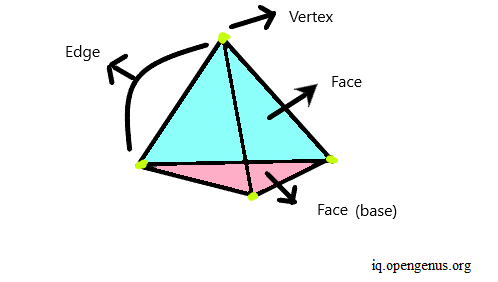This polyhedron has four faces-bottom, front, left and right. In terms of vertices, there is one common vertex shared by three faces at the top. We also have three more vertices, created when the three faces share an edge with the bottom face. This gives us four vertices. In terms of edges, there are three at the bottom and we get three when the side faces join with each other, using a common edge along the side. So, in total, we get six edges.

Applying Euler's formula, we get -

4+4-6 = 2,

which is as per the result we would expect from the formula.

### Octahedron

Another example to consider is an Octahedron.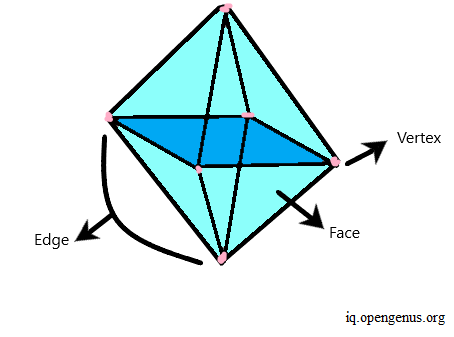In this Polyhedron, we have four triangular faces, built on top of a square base in the middle. On the bottom of this square base, we also have four more triangular faces below it. So, we can think of the square base in the middle that helps us to join the four top faces with the four bottom faces.

In actuality, the square base is an illusion, that appears when we join the four top faces using one shared edge, at each side, with the bottom four faces. So, we have in total, eight faces.

In terms of vertices, we have one vertex at the top, shared by the top four faces. Likewise, we have one vertex at the bottom. In addition, there are four more vertices in the middle, created when we join the four faces, using an edge for each face, with the bottom four faces. In total, we get six vertices.

In terms of edges, we have four edges, created when the four faces at the top join each other using a shared edge. Likewise, we have four edges for the bottom faces. We also get four more edges, when we join the top four faces with the bottom four faces. In totality, we get 12 edges.

Applying Euler's formula, we get-
8+6 - 12 = 2
This result is satisfactory as per Euler's formula.

### Dodecahedron

We can also look at the case of a Dodecahedron. This Polyhedron contains pentagonal faces.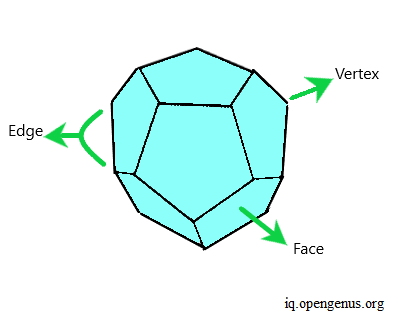We have one pentagonal face at the bottom, with five more such faces built on top of it. In addition, we then have five more faces that share an edge with the five bottom pentagonal faces. Then, at the top, we have one more pentagonal face. This gives us 12 pentagonal faces in total.

In terms of vertices, we have five vertices at the bottom. As we go to the middle, we get ten more vertices. Considering each pentagonal face, we have five joined with the bottom pentagonal face, and five faces built on top of these five faces. Towards the top, we get five more vertices. This in total, gives us 20 vertices.

When it comes to edges, we have five at the bottom. Then, we get five more edges, as five pentagonal faces built on top of the bottom share one edge with each other, considering from the bottom face. These five faces then share two edges each, with the other five faces built on top of each of them. So, this gives us ten more edges. The pentagonal faces built on top of the five pentagonal faces in the lower half, share one edge with each other. This gives us five more edges. At the top, we have another pentagonal face, which gives us five more edges. So, in total, we have thirty edges.

Applying Euler's formula, we have-
12 + 20 - 30 = 2
This result once again proves Euler's formula.

# Euler’s Characteristic

As mentioned in the introduction, the Euler's formula produces a result of two only in the case of convex polyhedrons. However, there are many 3-d surfaces where the result is not always two, but we can still make use of result from the Euler’s formula. We call the result of the Euler’s formula as Euler’s characteristic, denoted by χ. The formula is shown below.

Χ = V – E + F

As an extension of the two formulas discussed so far, mathematicians found that the Euler's characteristic for any 3d surface is two minus two times the number of holes present in the surface.

Χ = 2-2g, where g stands for the number of holes in the surface.

# Mathematical Proof and converting into Network

We prove Euler's theorem using mathematician Cauchy's method. In this method, we convert the polyhedron into a network. To convert the polyhedron into a network for our proof, we first remove the top face from the cube. Then we flatly lay down each of the remaining faces, ensuring that they still remain connected to each other as shown in the image below.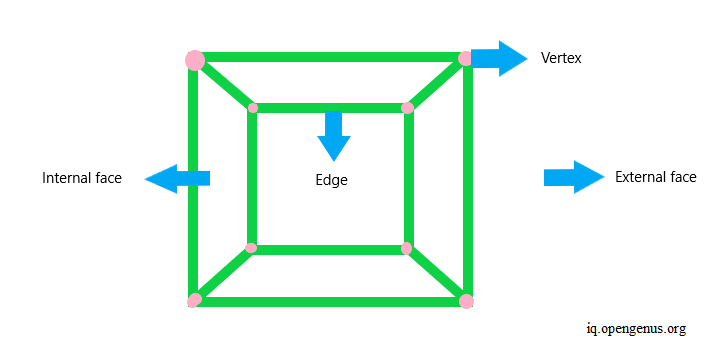The face that we remove becomes the external face and the rest of the internal faces still count as internal faces. The external face is the area surrounding the network. So, in totality, we still have the same number of faces (including the external face), edges and vertices.

We begin by ensuring that there are no faces with more than three edges. If there is such a face, we divide the face into further triangular faces using a diagonal.
We continue to repeat this step until there are no such faces, as shown below.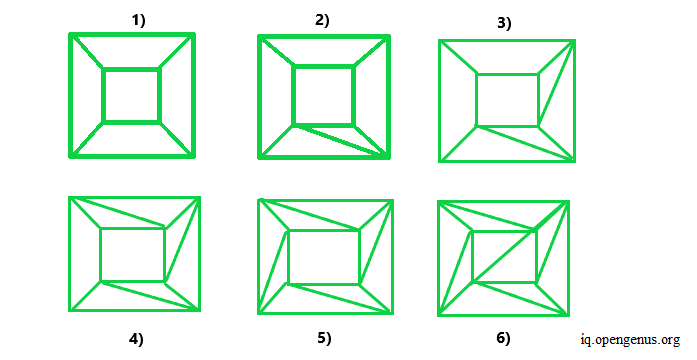So, at each step, we have one more face, one more edge and the number of vertices stays the same at every step. Therefore, using Euler’s formula, we get-

F + 1 + V – (E + 1) = F + V + 1 – E – 1 = F + V – E

At each stage of step one, we observe that Euler’s formula always holds till the end. This is shown in the image below.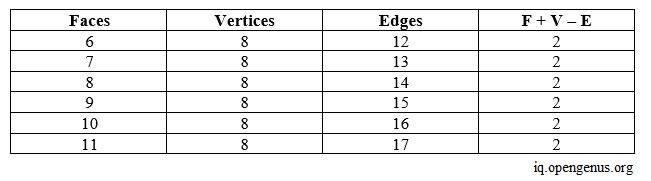Therefore, the result still holds as per the formula.

In step two, we start removing faces if they share one edge with the external face. We continue to remove such faces, till we no longer have them in our network. We can observe this at each stage in the image below.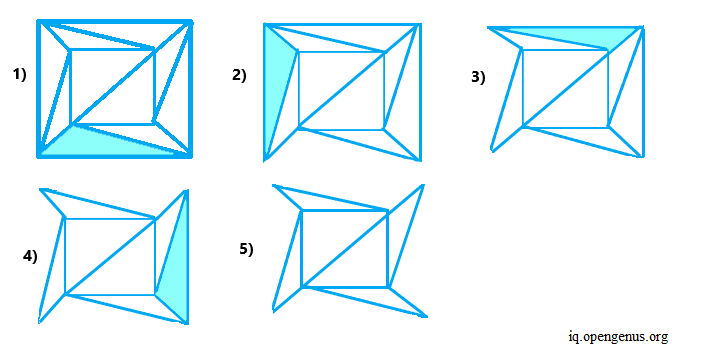In the final step, we remove each face that shares two edges with the external face. We continue to do this till all such faces are removed. During this step, we may also repeat step two, but only if in case there are no faces with two shared edges with the external face. We continue doing this, and in the end, get the final triangle as shown below.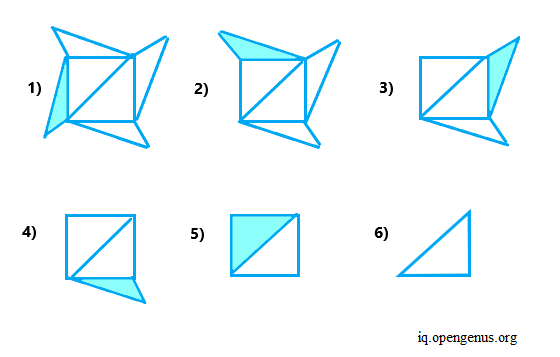As observed, in the very end, we get a network that has two faces, one internal and external, three edges and three vertices.

Applying the Euler's formula, we get-
3-3+2,
which gives us the result two as expected.

# Application of Euler’s Polyhedron Formula

Euler's formula is significant in graph theory, networking, and computer chip design.

• We use it to check whether a graph is a planar graph. A planar graph is a graph, which we can draw in a plane, in such a way so that no edges cross each other.

• Another application of Euler's formula is to check the connectivity of a graph. Connectivity in a graph requires that a path exists to reach any vertex from any other vertex.

• Also, we use Euler's formula for computer chip designing. Computer chips are much like circuits, containing several components. These chips have tracks that connect all the components in the computer chip. We can think of these circuits as graphs and the tracks as edges. When creating chips, it's vital that these tracks, or edges, don't intersect or cross each other. More generally, we must keep such intersections as low as possible. Thus, we use Euler's formula to find such solutions, where intersections are minimal.

With this article at OpenGenus, you must have the complete idea of Euler’s Polyhedron Formula.